# report final .pdf

### File information

Title: graphq4.eps
Author: scholar,,,

This PDF 1.4 document has been generated by gnuplot 4.2 patchlevel 6 / GPL Ghostscript 8.71, and has been sent on pdf-archive.com on 24/01/2014 at 13:27, from IP address 14.139.x.x. The current document download page has been viewed 1085 times.
File size: 4.61 MB (12 pages).
Privacy: public file

### Document preview

Computation of Tokamak Plasma Parameters Using the Function
Parametrization Technique
Pranav Gupta
Guided By:
Dr. Daniel Raju
Head, SST-1 Plasma Control Physics Division,
Institute for Plasma Research, Gandhinagar.

July 26, 2013
Abstract
The physical parameters needed for real-time plasma current, position and shape feedback control in a
tokamak are provided by an Inverse Mapping technique known as Function Parametrization. In this technique, analysis of Magnetohydrodynamics (MHD) equilibrium database using statistical methods is carried
out to evaluate various plasma parameters directly from diagnostic data. This avoids the computational
burden of repeatedly solving the tokamak equilibrium Grad-Shafranov equation in real time. The parameters so calculated provide the control algorithm with the actual values for the plasma current, position and
shape, whose deviations from pre-programmed (reference) values determine the control system response.
The control algorithm generally has a loop time of 100s of microseconds, while the control requirements are
1-10 ms. In this work, application of FP is performed on the database of first Indian Steady State Tokamak
(SST-1) and results are presented.

1

Introduction

Tokamak is a device that uses magnetic field to confine plasma in a torus-shaped configuration. To achieve this
equilibrium, magnetic field lines must wind helically around the torus. This can be done by establishing two
kinds of magnetic field intensities- poloidal (along the axis of symmetry of the torus) and toroidal (along the
circumference of the torus, in a tangential manner). The toroidal field (intensity) can be established by the
use of a solenoid-like configuration that winds the surface of the torus. Whereas the poloidal field is expected
to be generated by the plasma itself, when it moves around the torus. This, in turn, is done by changing
the magnetic flux density along the axis of symmetry of the torus, which drives a net plasma current around
the torus, according to Faraday’s law for electromagnetic induction. The use of magnetic fields for attaining
plasma equilibrium is necessitated by the fact that most materials cannot withstand plasma, making fully
material-based plasma confinement an extremely difficult option. Tokamaks hold a major promise towards the
achievement of sustainable nuclear fusion, which relies heavily on the control and confinement of reactants that
need to be in the plasma state at extremely high temperatures.
SST-1 (Steady State Superconducting Tokamak-1) is an Indian tokamak project aimed at the steady-state
operation of plasmas with advanced configurations. The toroidal magnetic field is supplied by superconducting
coils. Hydrogen gas acts as the source for generating plasma. Heating of plasma to desired temperatures is aided
by neutral beam injection (NBI), electron/ion cyclotron resonance heating (ECRH/ICRH) and radio frequency
(RF) waves. Additional coils for poloidal magnetic field are used in order to sustain plasma equilibrium and
the steady state. The diverter (a component that diverts charged particles from the plasma boundaries into a
chamber that collects and neutralizes them) has a double null configuration.
Plasma in a tokamak configuration experiences several instabilities that are primarily magneto-hydrodynamic
in nature. In order to sustain the plasma for a sufficient amount of time, one needs to apply additional controls
that counter-act such instabilities (for example, supplementary magnetic fields that control plasma position).
Control parameters are based on fast interpretation of real-time information from the plasma, in terms of its
shape, position, etc. This information has to be interpreted from diagnostic measurements, which generally
comprise of magnetic field intensity values from probes kept at different locations in the plasma. In other

1

Table 1: Some Important Parameters for SST-1
Value/Description
1.1 m
0.2 m
Toroidal Magnetic Field Intensity
3T
First Wall Material (material facing the plasma directly)
graphite-bolted tiles
Heating
1 MW (ICRH), 1 MW (NBI)
Parameter

words, we have to get a mapping from probe measurements to plasma parameters, which is an inverse of the
usual physical expression that evaluates probe measurements in terms of plasma parameters. In present-day
tokamaks, controlling the plasma position and other plasma parameters in a fast and efficient manner has been
one of the key goals, which asks for methods that can interpret data from plasma diagnostic measurements
and simultaneously calculate the plasma parameters. Function Parametrisation is one such procedure that is
widely used for serving the above-mentioned purpose.

2

Description of Function Parametrization (FP):

The method of function parametrization involves the following major steps:
1. Generation of a Database of simulated diagnostic measurements based on known parameters
2. Reducing the dimension of the database and conditioning it through methods like Principal Component
Analysis (PCA) and Latent Root Analysis
3. Regression to generate an approximate relation between parameters and diagnostic variables
The database is generated by taking different sets of values of independent parameters and computing the
corresponding set(s) of diagnostic measurements and other dependent parameters. In order to increase the
accuracy of predictions, the independent parameters are selected from Gaussian distributions that are peaked
at the most likely values of the plasma parameters and have spreads (or twice the standard deviations) that
are expected in an experimental situation. One must also ensure that the parameters do not take improbable
values, so that we can get rid of extremely unlikely plasma states that carry no physical significance. This
can be ensured by putting lower and upper limits on the values that the plasma parameters can assume. For
example, if area is an independent variable whose value is expected to be 1m2 during an experiment with a
spread of 0.2m2 , then the corresponding Gaussian distribution for selecting area value for the database must
be peaked at 1m2 , with a standard deviation of something like 0.1m2 .
The database thus generated is generally huge in size and may have several redundancies and collinearities;
hence we need to subject it to statistical procedures like PCA, which form the dimension reduction step. This
also saves computation times for further steps and helps in reducing the size and complexity of the database.
Principal Component Analysis (PCA): In PCA, linear combinations of data present in diagnostic variables
are chosen as principal components so as to select only those combinations which show significant variations
over several observations. One takes the covariance matrix of the appropriately scaled raw data. The orthonormalised eigenvector matrix of this positive, semi-definite matrix acts as the linear transformation that
transforms the data matrix into a matrix of principal components, that can be sorted by their variance. Some
of these principal components (generally with variances greater than some threshold value) are transferred on
to further steps. If the data has N observations and M probes, it can be stored in the form of an N × M
matrix, say A. Each entry in this matrix is then transformed by getting each entry subtracted by the mean of
the column to which it belongs, followed by dividing by the standard deviation of the same column. Hence we
get a new N × M matrix Q. In other words,
(j)−ai
,
qi (j) = aiσ(a
i)
where i, j vary from 1 to M , N respectively and qi (j), ai (j) denote the j th entries in the ith columns of Q, A
respectively.
ai , qi ; σ(ai ), σ(qi ) denote the means and standard deviations of the ith columns of A and Q respectively. The
covariance matrix of Q (Σ) can be defined in the following manner:
q .q
Σ(i, j) = Ni −1j , where notations have their usual meaning.

2

It is easy to notice that Σ is a real, symmetric M × M matrix. Hence, it has real eigenvalues, which sum up to
the trace of Σ, i.e., M .
The principal components are the vectors from the set {eT Q, where e is an eigenvector of the matrix Σ} which
show significant variance over the N available observations (beyond some threshold value). Equivalently, they
are the variables defined by e’s, which are essentially linear transformations of the set of probe measurements.
Further analysis is based on such principal components, rather than the actual raw data. Principal components
are divided by their corresponding eigenvalues of Σ before going to the regression step.
This process fulfils two objectives1. Reducing the complexity of the measurement space
2. Removing redundant information and collinearities from the raw data
The regression step involves a fit of the parameters with respect to diagnostic data that is modified in the
’dimension reduction’ step, mostly in terms of polynomial functions of these modified variables. The resultant
relation(s) is (are) then used to calculate parameters as functions of diagnostic measurements during the actual
measurements. The modified principal components (modified due to division by eigenvalues of Σ) are used for
this step. For a simple regression model where the parameter has a linear, multivariate polynomial expression
in terms of the principal components, we undergo the following procedure1. Suppose that the number of principal components is S. We construct an N × (S + 1) matrix, which has
the modified principal components as its first S columns. The last column is filled with 1’s.
2. If the vector of the corresponding N parameter measurements is denoted by x, then the vector of regression coefficients (c) has an expression as follows:
c = (P T P )−1 P T x (a),
x = c(1) ∗ p1 + c(2) ∗ p2 + ...... + c(S) ∗ pS + c(S + 1) (b),
where pi ’s are the principal components (or transformed variable values) derived from the probe measurements corresponding to the parameter x.
For a quadratic fit, we minimize the sum of the squares of the differences between the estimated parameter
values and the actual parameter values for the database, akin to linear fitting. The set of coefficients (d)
are obtained using an expression similar to (a), with the matrix P getting replaced by a real, symmetric
(S+2)∗(S+1)
× (S+2)∗(S+1)
matrix H (where S is the number of principal components). Also, the vector x gets
2
2
replaced by an (S+2)∗(S+1)
-component vector b. The expression for d is as follows:
2
d = (H T H)−1 H T b,
x = d(1) + d(2) ∗ p1 + d(3) ∗ p2 + ...... + d(S + 1) ∗ pS+1 + c(S + 1) +

P

i,j
0&lt;i≤S
i≤j

pi ∗ pj ∗ d(i ∗ S + 2 + j − i +

(i−1)∗(i−2)
),
2

where pi ’s are the principal components (or transformed variable values) derived from the probe measurements
corresponding to the test value of parameter x.

3

Application of FP to SST-1 Tokamak Plasma:

IPREQ computer code has been used to generate the database. This is an MHD equilibrium code which solves
the Grad-Shafranov equation using a few inputs in terms of the pressure and current profile parameters as well
as external poloidal field coil currents. The plasma position and shape parameters along with the magnetic fields
at the specified R and z locations1 are the output of the code. Database has been generated for 400 different
randomly distributed cases (as described in section 2) of plasma parameters. Both radial and axial magnetic
fields are computed at 24 locations so as to make the total of 48 simulated magnetic probe measurements.
FP was used to evaluate the linear and quadratic regression coefficients for three parameters-plasma current
* plasma inner radius2 , plasma current * plasma outer radius3 and plasma current * plasma centroid position4 ,
which can then be divided by the available plasma current values to get plasma inner radius, plasma outer
radius and plasma centroid position respectively.
Results and Error Estimates: In order to check the validity of the regression models, the parameter5 values
1 usual

from the axis of symmetry where the plasma begins
3 distance from the axis of symmetry where the plasma ends
4 distance of the centroid (point of peak plasma density, temperature) of the plasma from the axis of symmetry
5 From now on, plasma current, plasma inner radius, plasma outer radius and plasma centroid position shall be denoted by I ,
p
Ri , Ro and Rc respectively
2 distance

3

calculated using the regression model were plotted against their actual values (from the database) by adding
varying percentages of Gaussian noise to the raw data of probe measurements on a scatter plot.
Figure 1: Calculated Ip *Ri (A*m units) v/s Estimated Ip *Ri (A*m units) [linear]

180000

180000

160000

160000

140000

140000

120000

120000

100000

100000
100000

120000

140000

160000

180000

180000

180000

160000

160000

140000

140000

120000

120000

100000

120000

140000

160000

100000

120000

140000

160000

120000

140000

160000

180000

100000

100000
100000

120000

140000

160000

180000

180000

180000

160000

160000

140000

140000

120000

120000

100000

180000

100000
100000

120000

140000

160000

180000

100000

200000

200000

180000

180000

160000

160000

140000

140000

120000

120000

100000

180000

100000
100000

120000

140000

160000

180000

200000

200000
180000
160000
140000
120000
100000
100000

120000

140000

160000

180000

200000

100000
200000
180000
160000
140000
120000
100000
80000
80000

120000

100000

120000

140000

140000

160000

160000

180000

180000

200000

200000

Table 2: Percentage Root Mean Square Error Values-1
Percentage Error
1
2
3
4
5
6
7
8
9
10

Value (Linear Regression) [in A*m units]

1.00
1.48
1.96
2.64
3.05
3.82
4.42
4.75
5.45
5.97

Value (Quadratic Regression) [in A*m units]

0.63
1.15
1.64
2.32
2.83
3.60
3.83
4.79
5.14
5.31

4

Figure 2: Calculated Ip *Ri (A*m units) v/s Estimated Ip *Ri (A*m units) [quadratic]

180000

180000

160000

160000

140000

140000

120000

120000
100000

100000
100000

120000

140000

160000

180000

100000

180000

180000

160000

160000

140000

140000

120000

120000

100000

120000

140000

160000

180000

100000
100000

120000

140000

160000

180000

180000

180000

160000

160000

140000

140000

120000

120000

100000

100000

120000

140000

160000

180000

100000

120000

140000

160000

180000

100000

120000

140000

160000

180000

100000
100000

120000

140000

160000

180000
200000

180000

180000

160000

160000

140000

140000

120000

120000

100000

100000
100000

120000

140000

160000

180000

180000

180000

160000

160000

140000

140000

120000

120000

100000

200000

100000
100000

120000

140000

160000

180000

100000

120000

140000

160000

180000

Figure 3: Calculated Ip *Ro (A*m units) v/s Estimated Ip *Ro (A*m units) [linear]
280000
260000
240000
220000
200000
180000
160000
140000

280000
260000
240000
220000
200000
180000
160000
140000
140000 160000 180000 200000 220000 240000 260000 280000

280000
260000
240000
220000
200000
180000
160000
140000

140000 160000 180000 200000 220000 240000 260000 280000
280000
260000
240000
220000
200000
180000
160000
140000

140000 160000 180000 200000 220000 240000 260000 280000
280000
260000
240000
220000
200000
180000
160000
140000
140000 160000 180000 200000 220000 240000 260000 280000
280000
260000
240000
220000
200000
180000
160000
140000
120000

140000 160000 180000 200000 220000 240000 260000 280000
280000
260000
240000
220000
200000
180000
160000
140000
120000
120000 140000 160000 180000 200000 220000 240000 260000 280000
280000
260000
240000
220000
200000
180000
160000
140000

120000 140000 160000 180000 200000 220000 240000 260000 280000

140000 160000 180000 200000 220000 240000 260000 280000

140000 160000 180000 200000 220000 240000 260000 280000

280000
260000
240000
220000
200000
180000
160000
140000
120000
120000 140000 160000 180000 200000 220000 240000 260000 280000

280000
260000
240000
220000
200000
180000
160000
140000

5

Figure 4: Calculated Ip *Ro (A*m units) v/s Estimated Ip *Ro (A*m units) [quadratic]
280000
260000
240000
220000
200000
180000
160000
140000

280000
260000
240000
220000
200000
180000
160000
140000
140000 160000 180000 200000 220000 240000 260000 280000
280000
260000
240000
220000
200000
180000
160000
140000

140000 160000 180000 200000 220000 240000 260000 280000
280000
260000
240000
220000
200000
180000
160000
140000

140000 160000 180000 200000 220000 240000 260000 280000
280000
260000
240000
220000
200000
180000
160000
140000
140000 160000 180000 200000 220000 240000 260000 280000
280000
260000
240000
220000
200000
180000
160000
140000
120000

140000 160000 180000 200000 220000 240000 260000 280000
280000
260000
240000
220000
200000
180000
160000
140000
120000
120000 140000 160000 180000 200000 220000 240000 260000 280000
280000
260000
240000
220000
200000
180000
160000
140000

120000 140000 160000180000 200000 220000240000 260000 280000
280000
260000
240000
220000
200000
180000
160000
140000
140000 160000 180000 200000 220000 240000 260000 280000

140000 160000 180000 200000 220000 240000 260000 280000
280000
260000
240000
220000
200000
180000
160000
140000
120000
120000 140000 160000 180000 200000 220000 240000 260000 280000

Table 3: Percentage Root Mean Square Error Values-2
Percentage Error
1
2
3
4
5
6
7
8
9
10

Value (Linear Regression) [in A*m units]

0.96
1.35
1.99
2.42
2.89
3.64
4.06
4.47
5.53
5.46

Value (Quadratic Regression) [in A*m units]

0.56
1.14
1.74
2.27
2.84
3.44
3.97
4.29
5.10
5.61

6

Figure 5: Calculated Ip *Rc (A*m units) v/s Estimated Ip *Rc (A*m units) [linear]
240000
220000
200000
180000
160000
140000
120000

220000
200000
180000
160000
140000
120000
120000

140000

160000

180000

200000

220000

120000

140000

160000

180000

200000

220000

200000

220000

240000

240000
220000
200000
180000
160000
140000
120000

220000
200000
180000
160000
140000
120000
120000

140000

160000

180000

200000

220000

120000

240000
220000
200000
180000
160000
140000
120000
120000

140000

160000

180000

200000

220000

240000

220000
200000
180000
160000
140000
120000
100000
100000

120000

140000

140000

160000

160000

180000

180000

200000

240000

220000

240000
220000
200000
180000
160000
140000
120000

240000
220000
200000
180000
160000
140000
120000
120000

140000

160000

180000

200000

220000

240000

120000

140000

160000

180000

200000

220000

240000

260000
240000
220000
200000
180000
160000
140000
120000

240000
220000
200000
180000
160000
140000
120000
120000

140000

160000

180000

200000

220000

240000

120000 140000 160000 180000 200000 220000 240000 260000

7

Figure 6: Calculated Ip *Rc (A*m units) v/s Estimated Ip *Rc (A*m units) [quadratic]
240000
220000
200000
180000
160000
140000
120000

220000
200000
180000
160000
140000
120000
120000

140000

160000

180000

200000

220000

120000

240000
220000
200000
180000
160000
140000
120000

140000

160000

180000

200000

220000

200000

220000

240000

240000
220000
200000
180000
160000
140000
120000
120000

140000

160000

180000

200000

220000

240000

240000
220000
200000
180000
160000
140000
120000
120000

140000

160000

180000

200000

220000

240000

120000

220000
200000
180000
160000
140000
120000
100000
100000

120000

140000

140000

160000

160000

180000

180000

200000

240000

220000

240000
220000
200000
180000
160000
140000
120000

240000
220000
200000
180000
160000
140000
120000
120000

140000

160000

180000

200000

220000

240000

120000

140000

160000

180000

200000

220000

240000

260000
240000
220000
200000
180000
160000
140000
120000

240000
220000
200000
180000
160000
140000
120000
120000

140000

160000

180000

200000

220000

240000

120000 140000 160000 180000 200000 220000 240000 260000

Table 4: Percentage Root Mean Square Error Values-3
Percentage Error
1
2
3
4
5
6
7
8
9
10

Value (Linear Regression) [in A*m units]

1.02
1.55
2.02
2.79
3.12
3.89
4.49
4.74
5.38
6.05

Value (Quadratic Regression) [in A*m units]

0.60
1.09
1.72
2.23
2.95
3.34
4.11
4.41
4.83
5.56

8

4

Concluding Remarks and Future Work:

The scatter plots and root mean square error estimates indicate that quadratic regression has a slight edge over
linear regression in terms of accuracy. But quadratic fitting is time-intensive. Generally there is a trade-off
between faster computation time and accuracy. The choice of method depends upon the need and situation.
Also, the database can be generated by computer simulations of the experiment in a software like COMSOL
Multiphysics, apart from the IPREQ code used here. They use finite-element methods to numerically solve
the physical equations connecting plasma parameters and probe measurements. Use of such a software has an
The above-mentioned work does not explain the later steps that complete the feedback-cum-control loop.
In these steps, one basically uses equations (b) and (d) to compute plasma parameters in terms of principal
components6 derived from the test probe measurements after appropriately combining them with the regression
coefficients, as per the regression model (linear, quadratic, etc.).The differences between computed parameter
values and some fixed, reference values (for each parameter) are calculated. From this information, one gets
the required value(s) for control variable(s) (for example, correction current) through a linear transformation
of parameter values.

5

References:
1. van Milligen et al., Analysis of Equilibrium and Topology of Tokamak Plasmas (Rijnhuizen Report 91-205)
2. Collins and Chatfield, Introduction to Multivariate Analysis
3. Wikipedia.org (articles on SST-1 and Tokamak)
4. Description of SST-1 from www.ipr.res.in
5. Braams et al., Fast Interpretation of Plasma Parameters, Nuclear Fusion, Vol. 26, 1986
Appendix:MATLAB/Octave Codes for Regression

Linear Fit:
function princomp ( )
format l o n g ;
K=400;
q=load ( ’ e x t r a c t . d a t ’ ) ( 1 : K , : ) ;
raw=q ;
f o r j =1:48
q ( : , j )=( q ( : , j ). −mean( q ( : , j ) ) ) . / std ( q ( : , j ) ) ;
end
f o r k =1:48
f o r j =1:48
sigma ( k , j )=q ( : , k ) ’ ∗ q ( : , j ) ;
sigma ( k , j )= sigma ( k , j ) / (K− 1 ) ;
end
end
[ EVEC, EVAL, F]=svd ( sigma ) ;
EVEC=EVEC ( : , 1 : 8 ) ;
pc=q∗EVEC;
f o r j =1:8
pcn ( : , j )=pc ( : , j ) . /EVAL( j , j ) ;
end
pcn ( : , 9 ) = ones (K, 1 ) ;
f o r m=2:4
a=load ( ’ p a r f p n 2 . o u t ’ ) ( 1 : K,m) . ∗ load ( ’ p a r f p n 2 . o u t ’ ) ( 1 : K, 1 ) ;
c o e f f ( : ,m)=( pcn ’ ∗ pcn ) \ ( pcn ’ ∗ a ) ;
f o r l =1:10
mse ( l ) = 0 ;
end
f o r l =1:10
q1=raw ;
f o r k =1:K
f o r j =1:48
q1 ( k , j )=q1 ( k , j ) + 0 . 0 1 ∗ l ∗randn ( ) ∗mean( raw ( : , j ) ) / 3 ;
end
end
f o r j =1:48
q1 ( : , j )=( q1 ( : , j ). −mean( q1 ( : , j ) ) ) . / std ( q1 ( : , j ) ) ;
end
pc=q1 ∗EVEC;
f o r j =1:8
6 The

test raw data (if small in size) is initially transformed using the mean and standard deviations of the database columns

9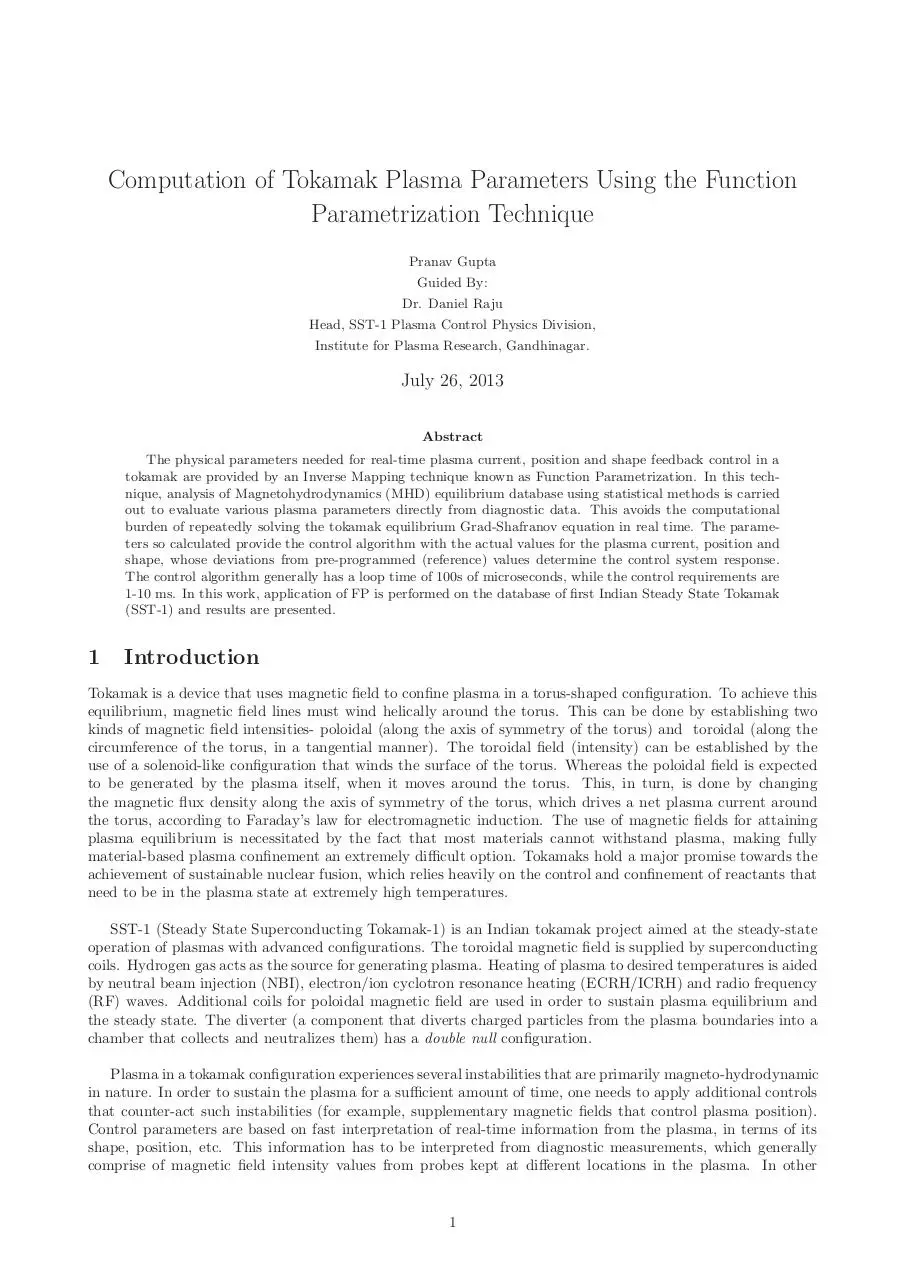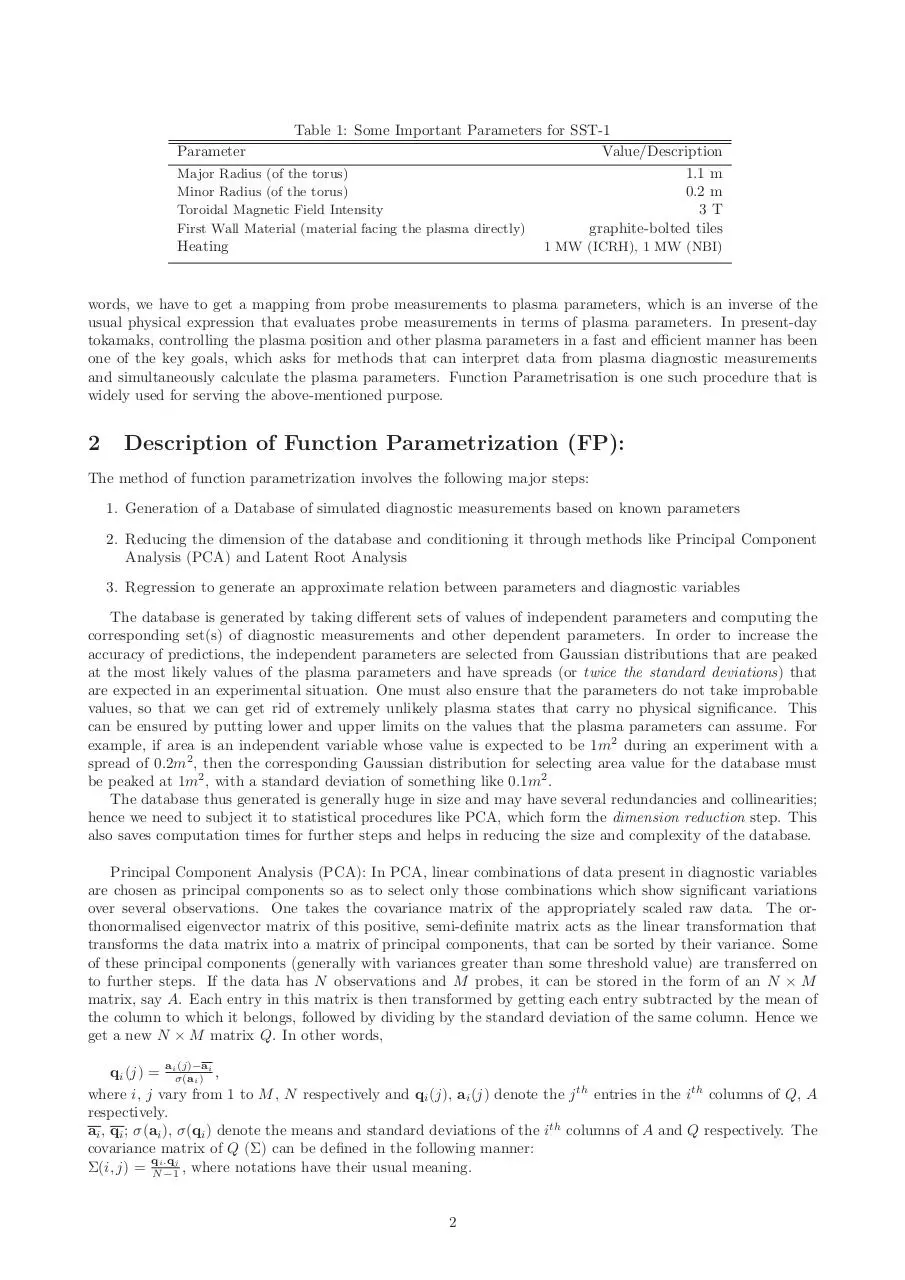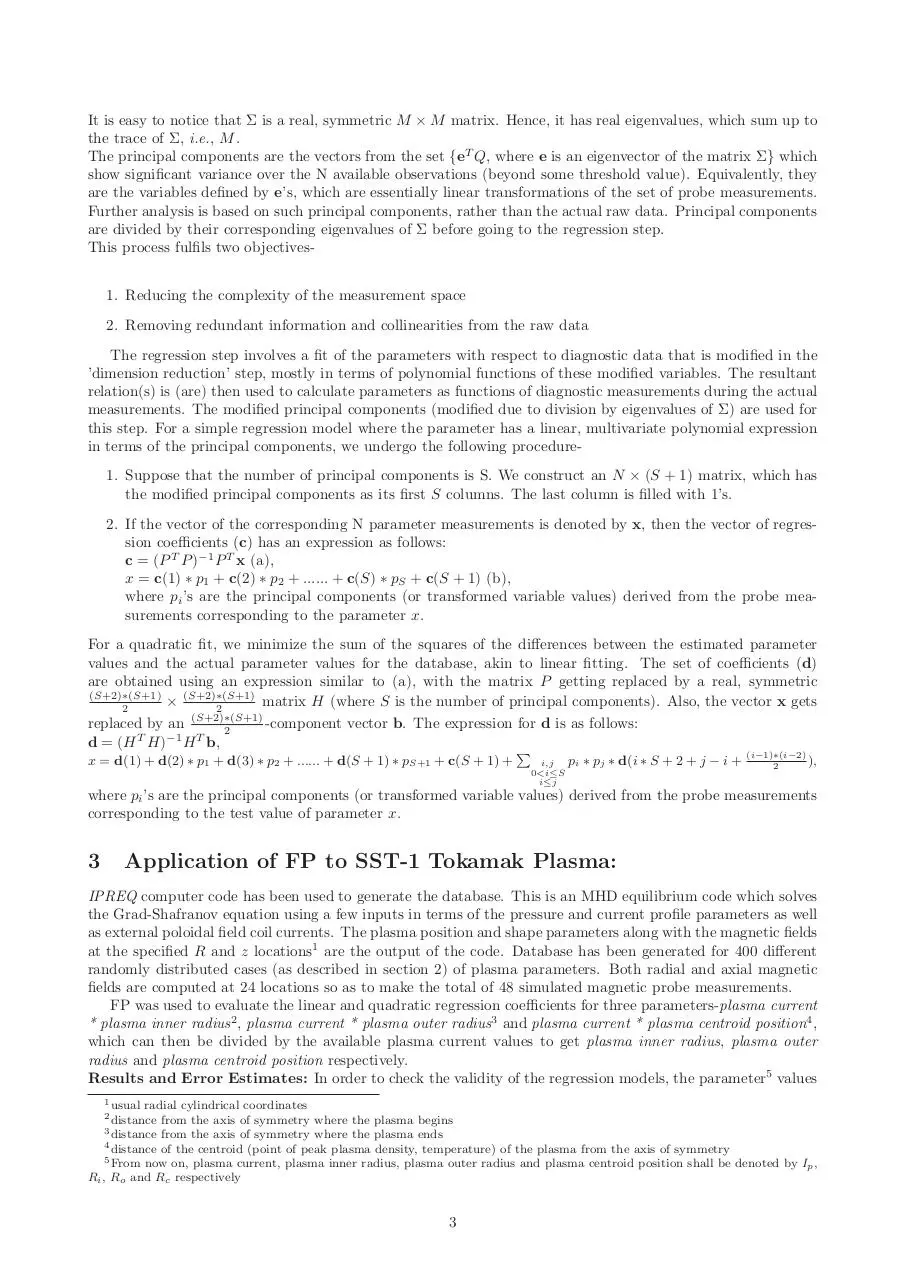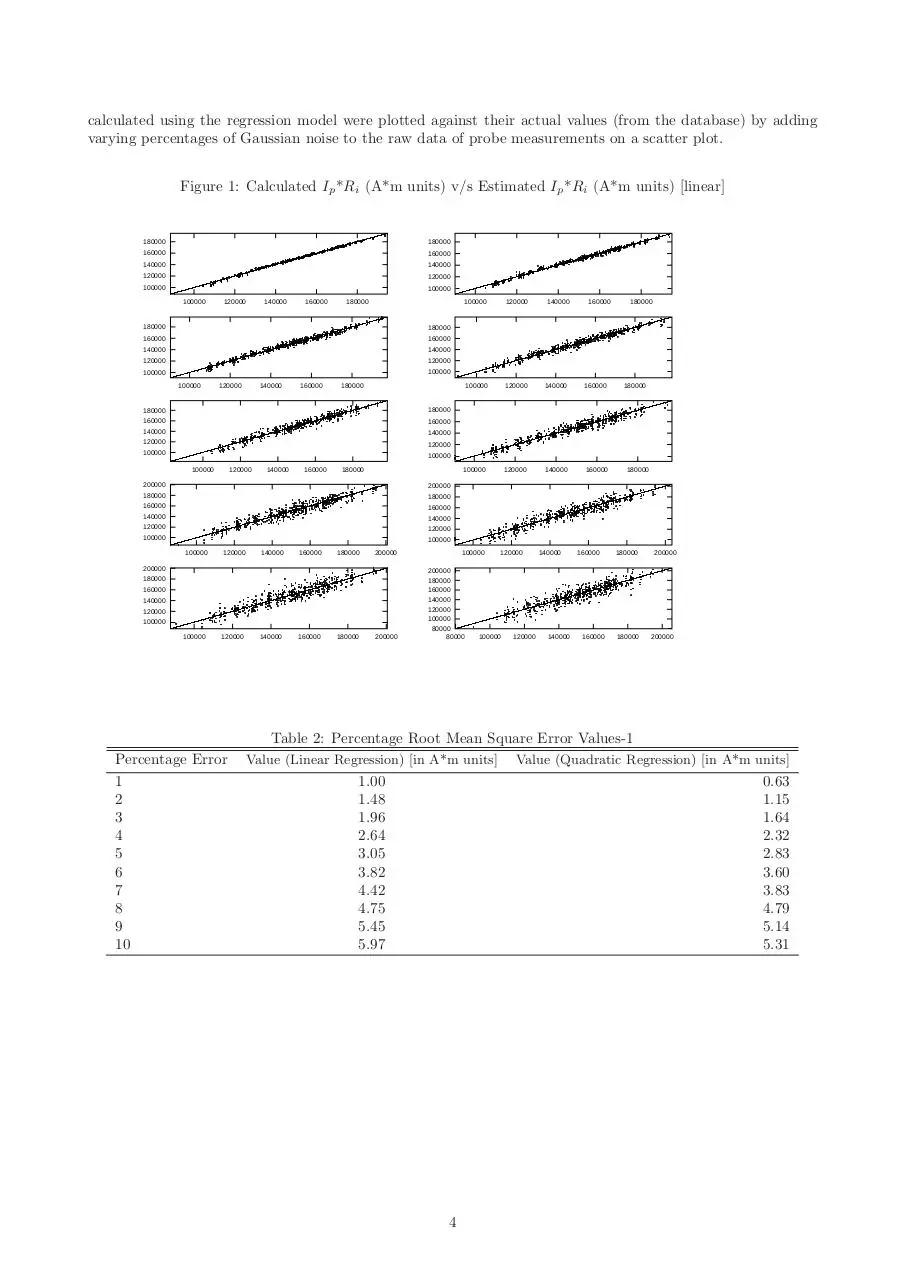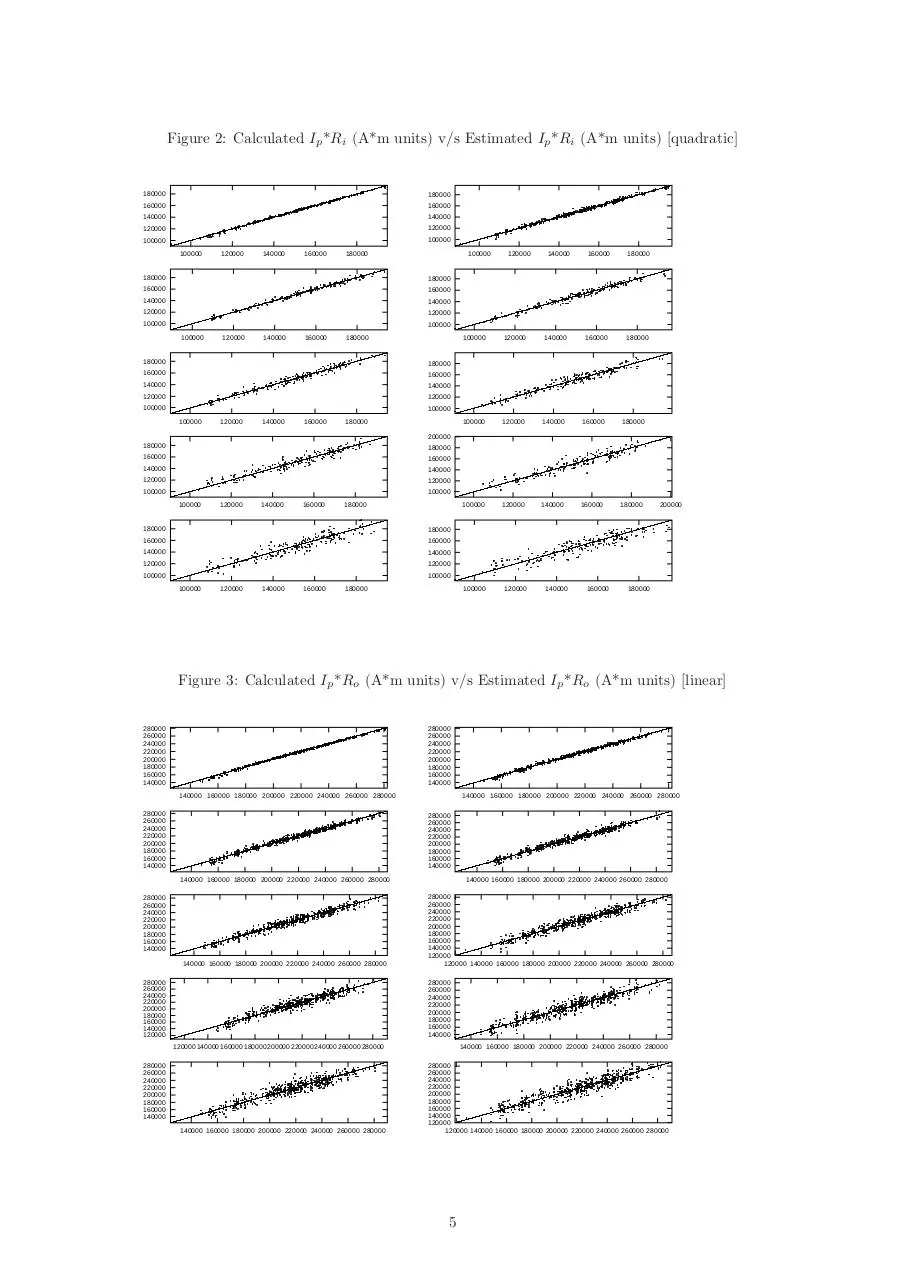report_final.pdf (PDF, 4.61 MB)

### Share on social networks

#### HTML Code

Copy the following HTML code to share your document on a Website or Blog